Function Repository Resource:

Compute the relative probability of finding a point at a given distance from another point

Contributed by: Nicholas E. Brunk, Wolfram|Alpha Math Team
 ResourceFunction["RadialDistributionFunctionList"][{p1,p2,…},L] returns the radial distribution function resulting from each pair of points pi1,pi2 within a periodic box of uniform dimensions L. ResourceFunction["RadialDistributionFunctionList"][{p1,p2,…},L,w] returns the radial distribution function using separation bin width w.

## Details

The radial distribution function is also known as the pair correlation function.
The default value for the bin width (w = 0.005), is a common choice in reduced units quantified relative to the particle diameter.
ResourceFunction["RadialDistributionFunctionList"] will work in up to three dimensions, applying periodicity uniformly in each.
ResourceFunction["RadialDistributionFunctionList"] normalizes the y axis to generate a density relative to that of an ideal gas at the same density in the same space (determined by box size L and the number and dimension of the specified coordinates).
ResourceFunction["RadialDistributionFunctionList"] takes the option "ReferenceCoordinates" {pj1,pj2,} to compute the RDF gij=gji between two pairs of coordinates {pi1,pi2,} and {pj1,pj2,}.
ResourceFunction["RadialDistributionFunctionList"] utilizes Compile for performance enhancement, and takes the same options.

## Examples

### Basic Examples (2)

Compute the radial distribution function from two points:

 In:=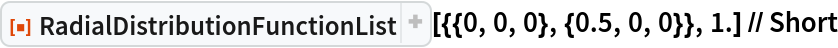Out=Compute the radial distribution function of a set of coordinates enclosed in a periodic 1D "box" of size (L = 4):

 In:=Display the coordinates in number line format along the axis for which the positions differ:

 In:=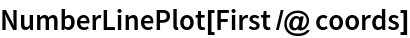Out=The radial distribution function has non-zero peaks at positions near those of the exact distances apart:

 In:=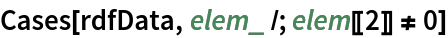Out=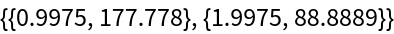Plot the radial distribution function characteristic of this system:

 In:=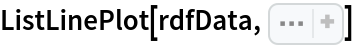Out=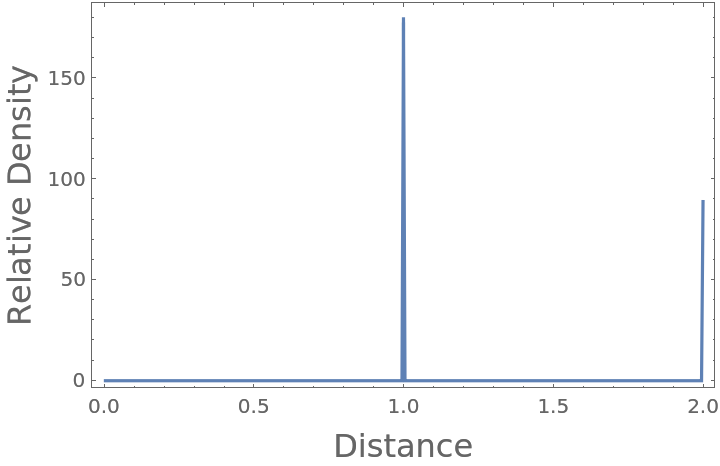### Options (2)

Cross-correlations can be computed using the option "ReferenceCoordinates":

 In:=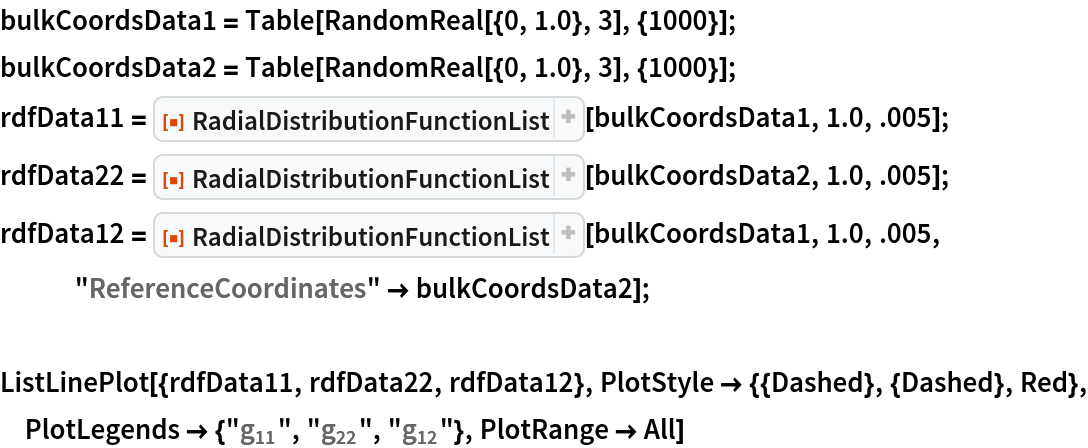Out=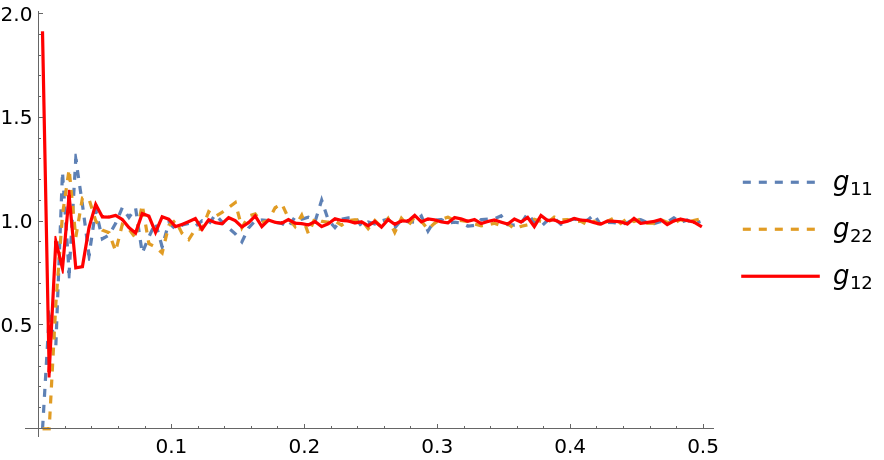Radial distribution function takes the same options as Compile, and benefits from automated compilation to C:

 In:=Out=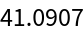In:=Out=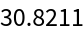### Applications (2)

Compute the radial distribution function characteristic of a cube with unit length, enclosed in a sufficiently larger periodic box:

 In:=Out=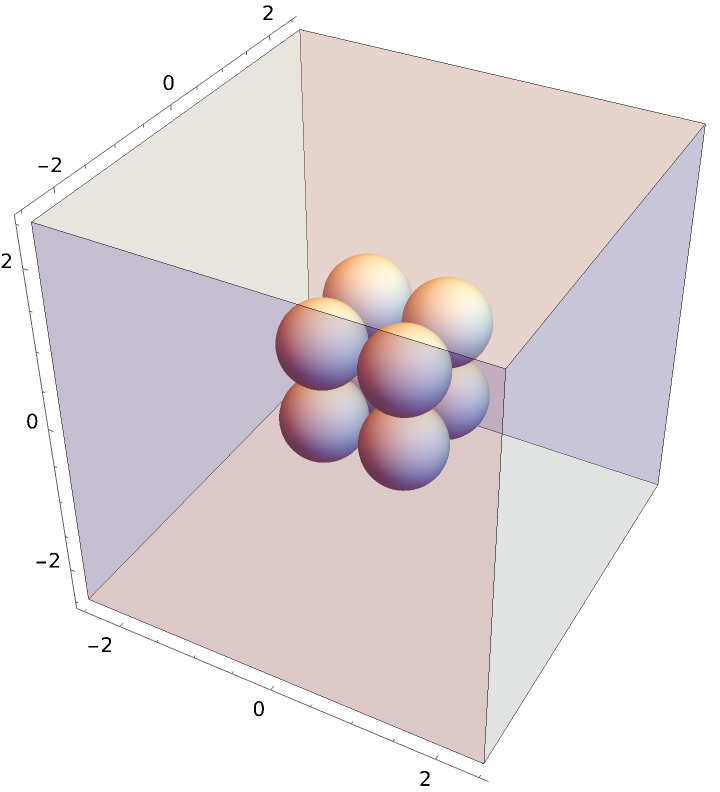Plot the resulting radial distribution function, where you can see nearest neighbor, polygonal face hypotenuse, and internal hypotenuse distances:

 In:=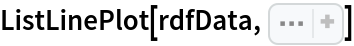Out=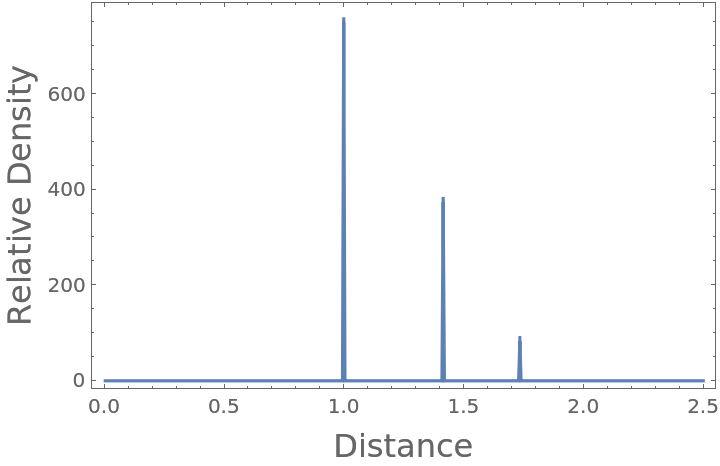### Properties and Relations (4)

By definition, the RDF of an ideal gas converges to 1 at all distances when provided sufficient data (here, 1000 time steps on 100 positions of 100 particles):

 In:=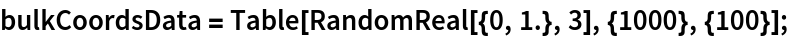Compute both the radial distribution function of just the first time step, and then that of the ensemble average over all time steps:

 In:=As expected, the radial distribution function converges to nearly 1 as sufficient data is introduced:

 In:=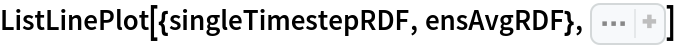Out=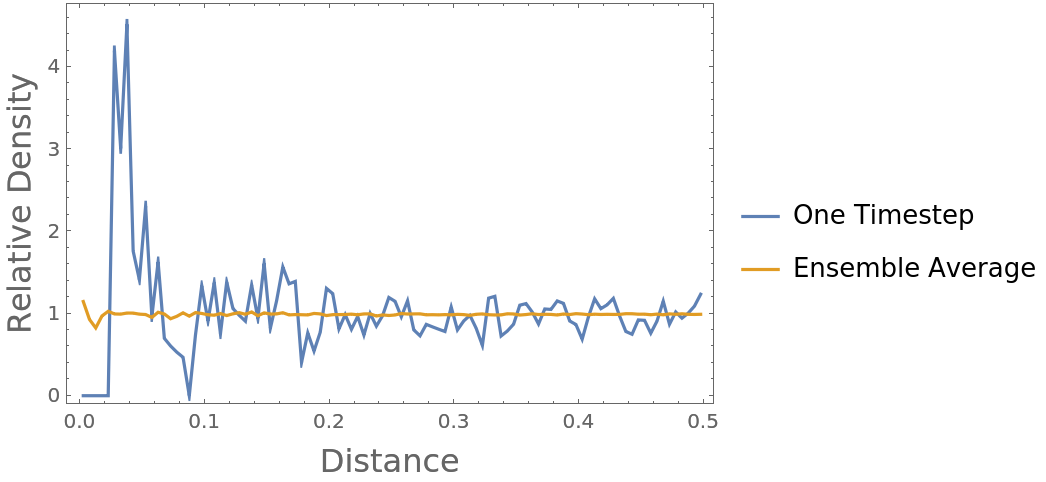Normalization by the expected number of particles in that vicinity works in 2D cases as well:

 In:=In:=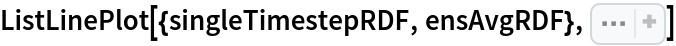Out=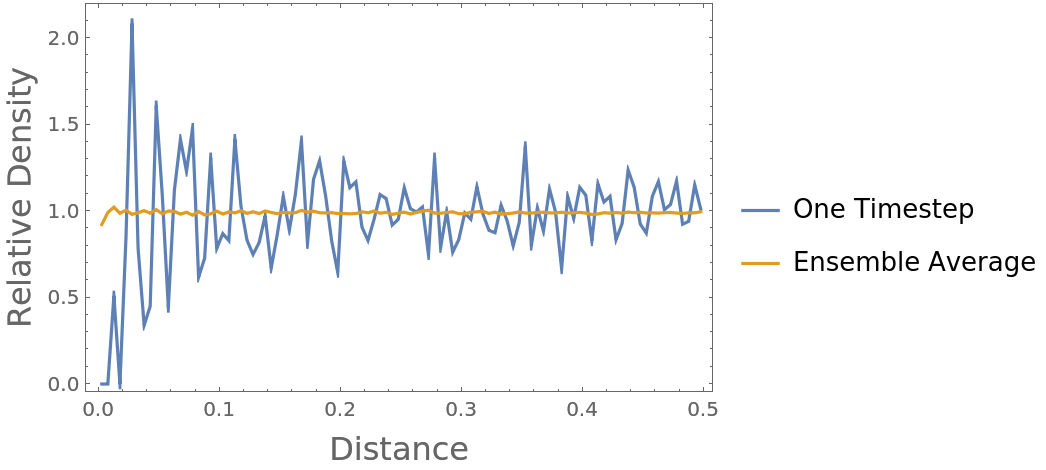The same is true for 1D cases:

 In:=In:=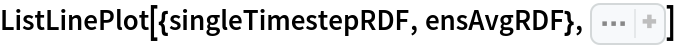Out=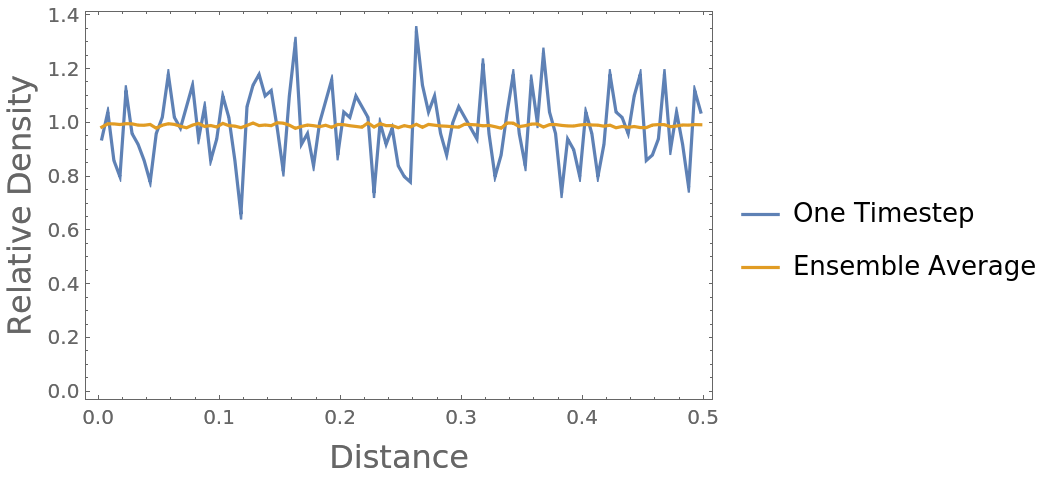Ensemble average cross-correlations between two distinct populations (lists of coordinates) can also be computed:

 In:=Out=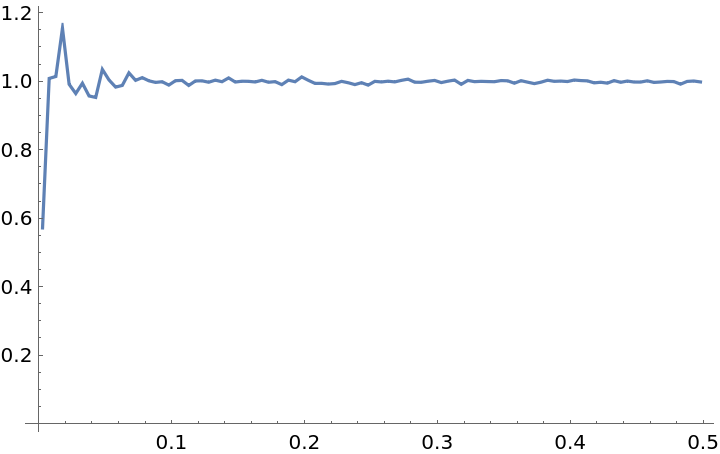Cross-correlations are symmetric, that is, the computation is the same for swapped populations g{XMLElement[i, {}, {XMLElement[span, {class -> stylebox}, {ij}]}]}(r)=g{XMLElement[i, {}, {XMLElement[span, {class -> stylebox}, {ji}]}]}(r):

 In:=Out=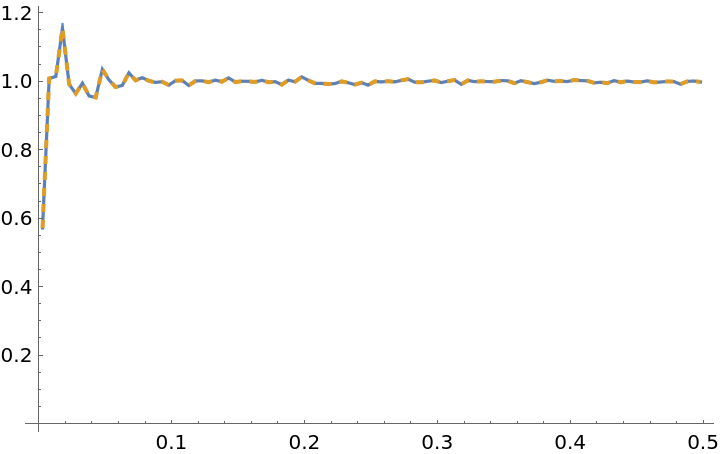In:=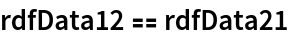Out=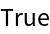### Possible Issues (4)

For finite-sized systems, the radial distribution function is accurate out to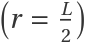and is thus truncated at this point, beyond which it would artificially decay:

 In:=Out=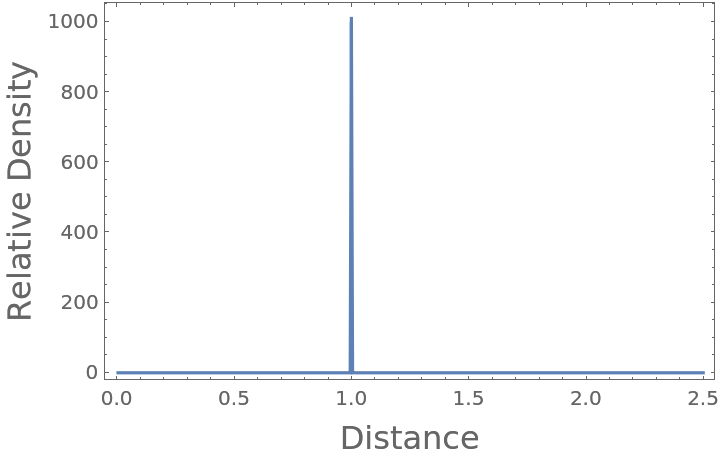If the box is sufficiently larger than the domain of the particle coordinates, the calculation is as expected (using real particle positions, rather than the minimum image convention under periodicity):

 In:=Out=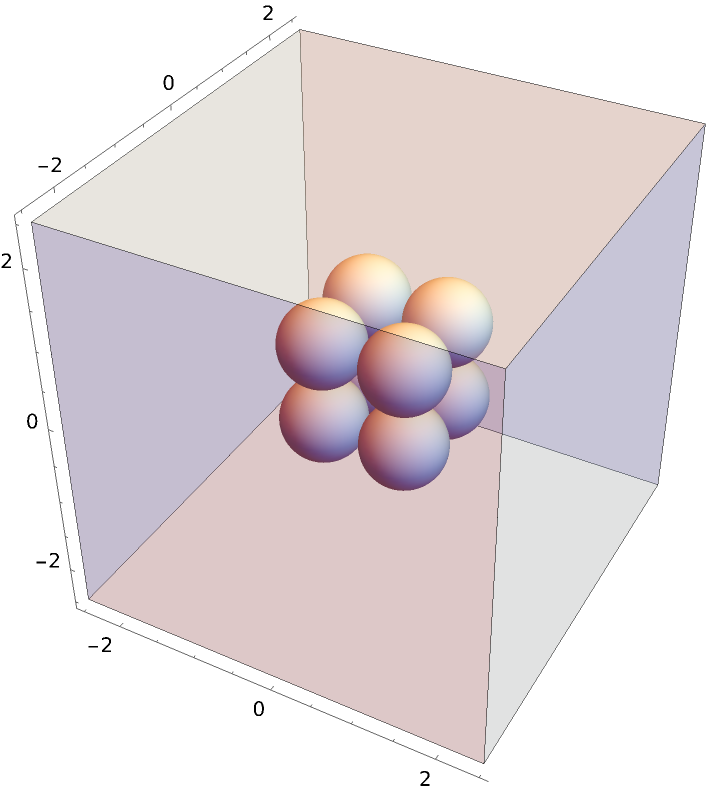The peaks are at the expected unit distance for the touching particles, at the polygonal face hypotenuse distance, and the interior hypotenuse:

 In:=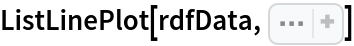Out=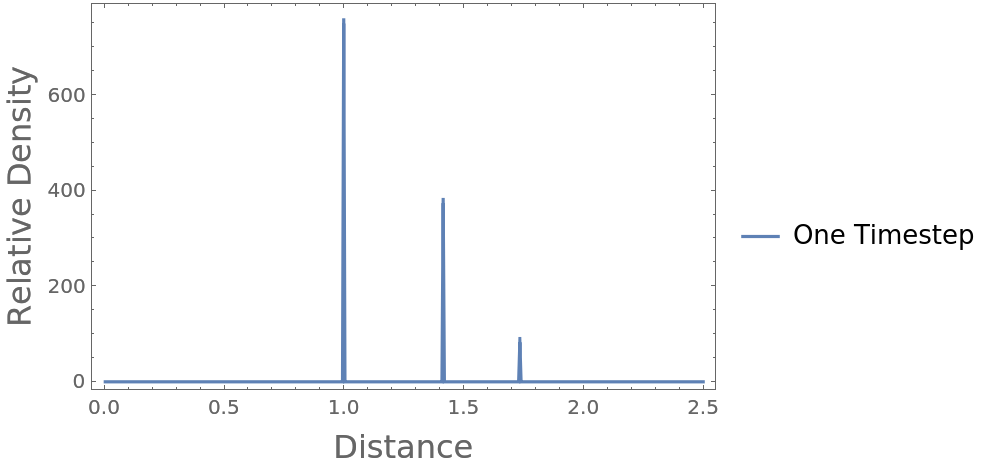If the box size (L) is too small, the minimum image convention is employed, applying periodicity in each dimension:

 In:=Out=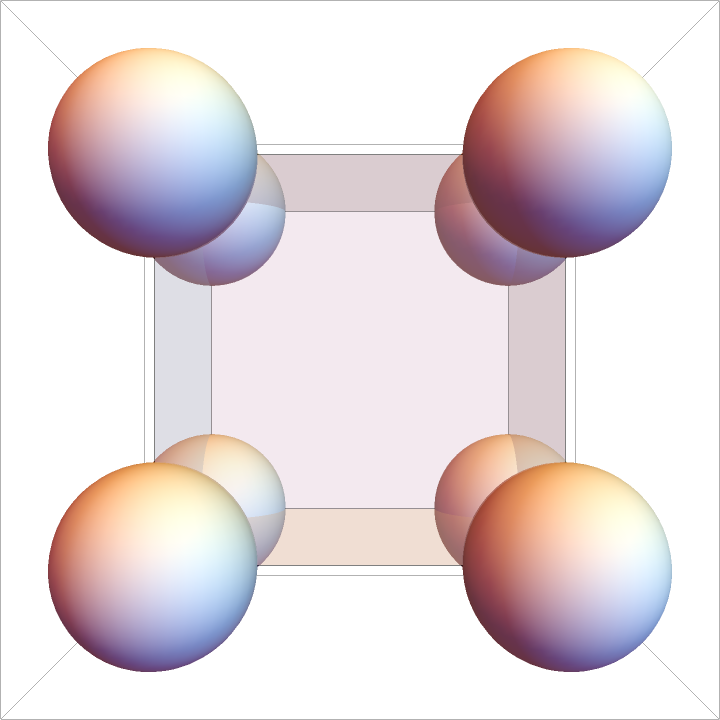In this case, particles are perfectly overlapping and the separation distance between all pairs is r{XMLElement[i, {}, {XMLElement[span, {class -> stylebox}, {ij}]}]}=0, however the convention is to put them in the first non-zero bin:

 In:=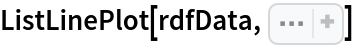Out=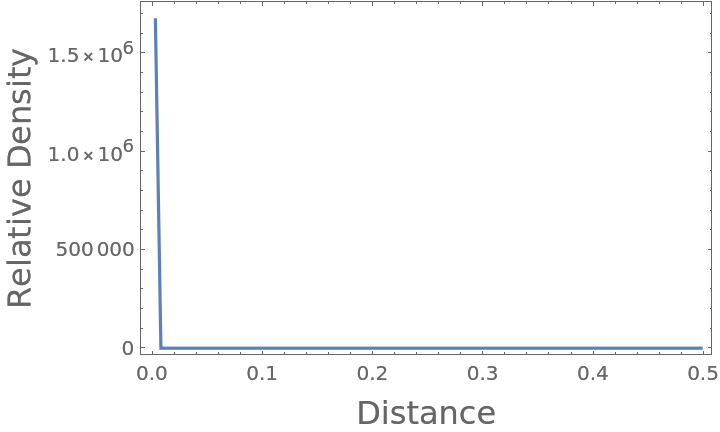Modifying the concentric shell bin width can affect normalization and positional accuracy, similar to the behavior in Riemann sums with too few discretizing rectangles:

 In:=Out=Compare the difference when using two different bin widths:

 In:=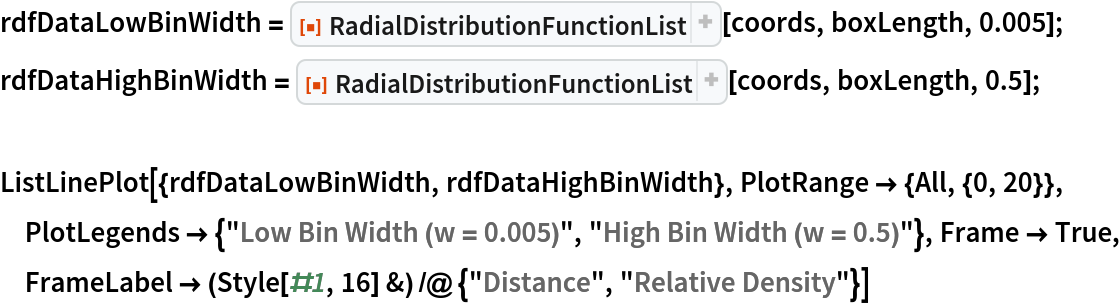Out=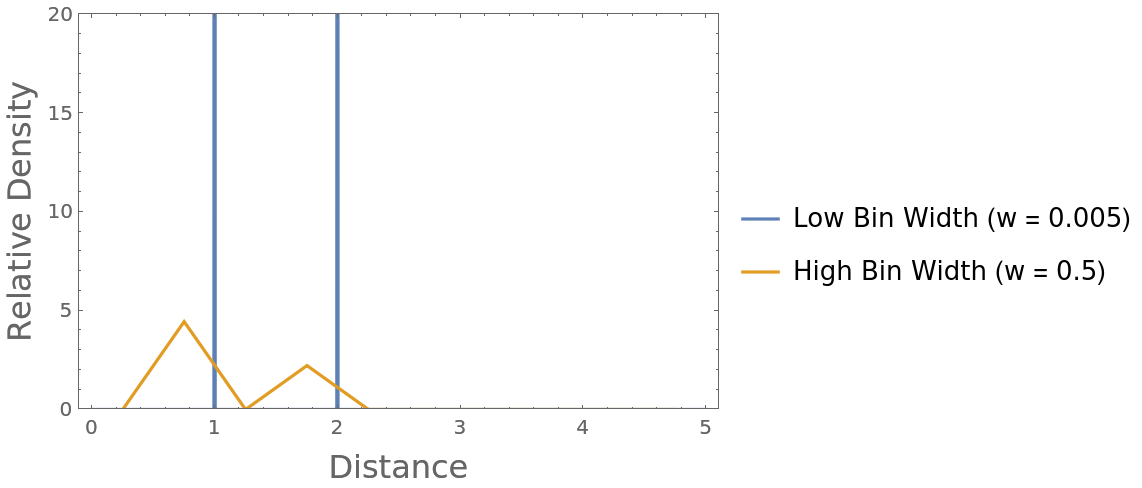### Neat Examples (3)

Compute the radial distribution function characteristic of an icosahedral distribution of particles and compare it with that of a cube, each with a minimum separation distance of 1:

 In:=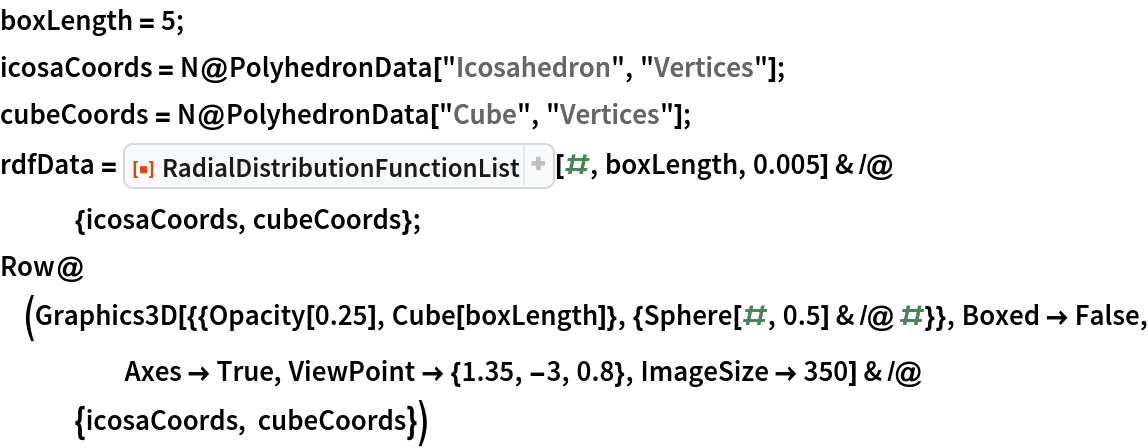Out=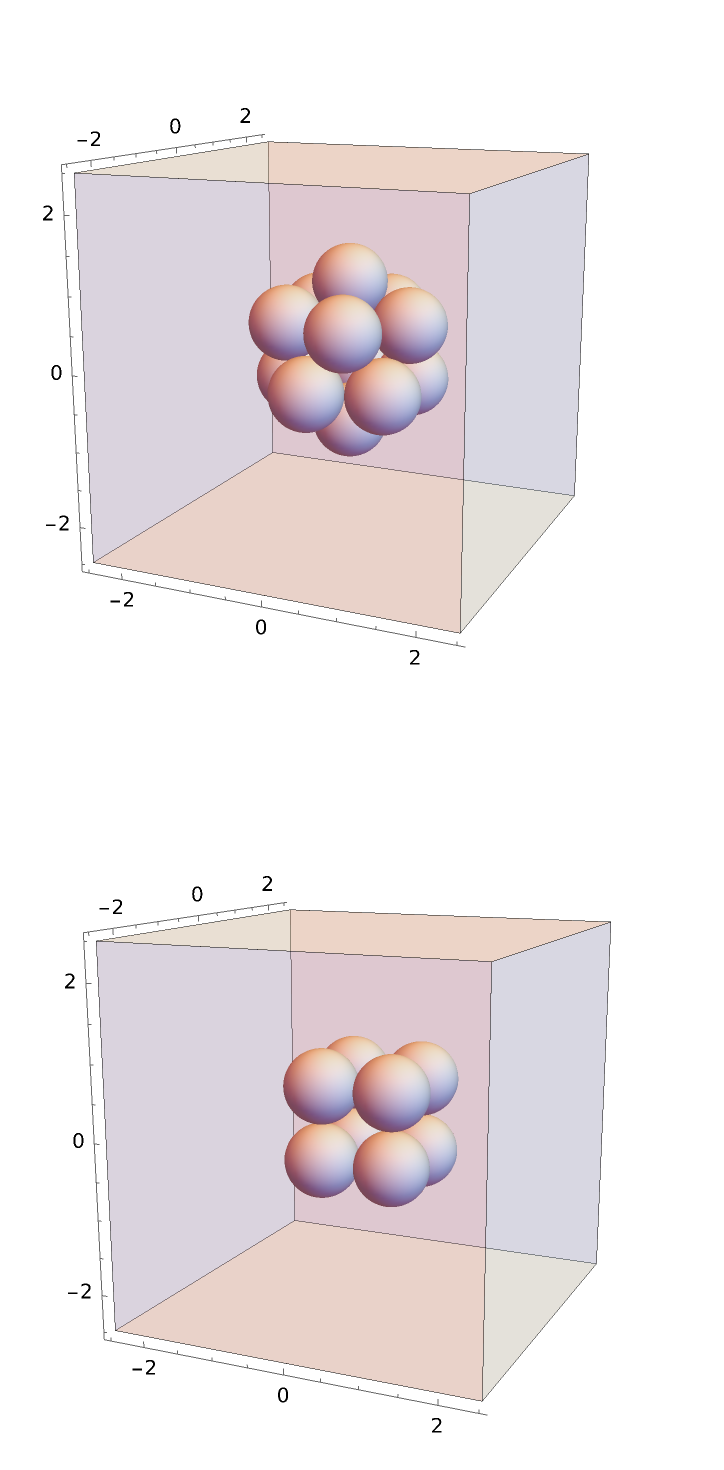Plot the resulting radial distribution functions to compare:

 In:=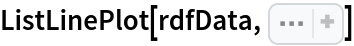Out=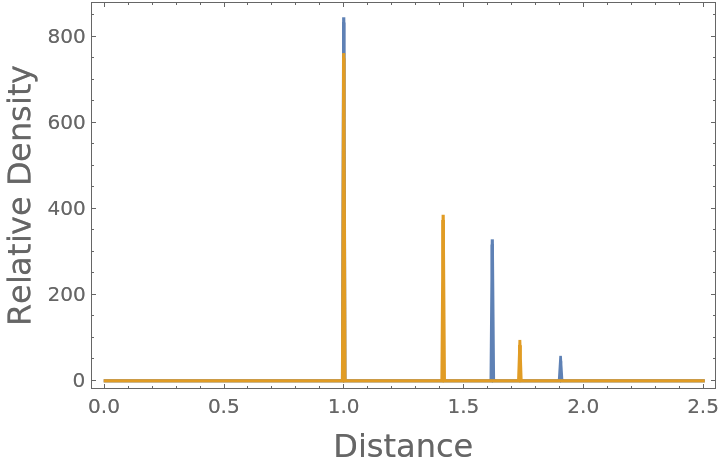Compare the radial distribution function of both a simple cubic and face-centered cubic (FCC) crystalline lattice (neglecting periodicity, each with nearest neighbors touching at unit lengths):

 In:=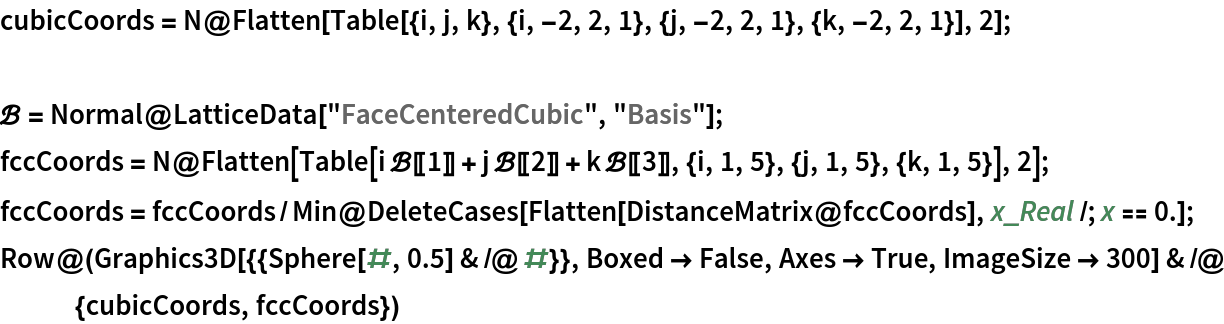Out=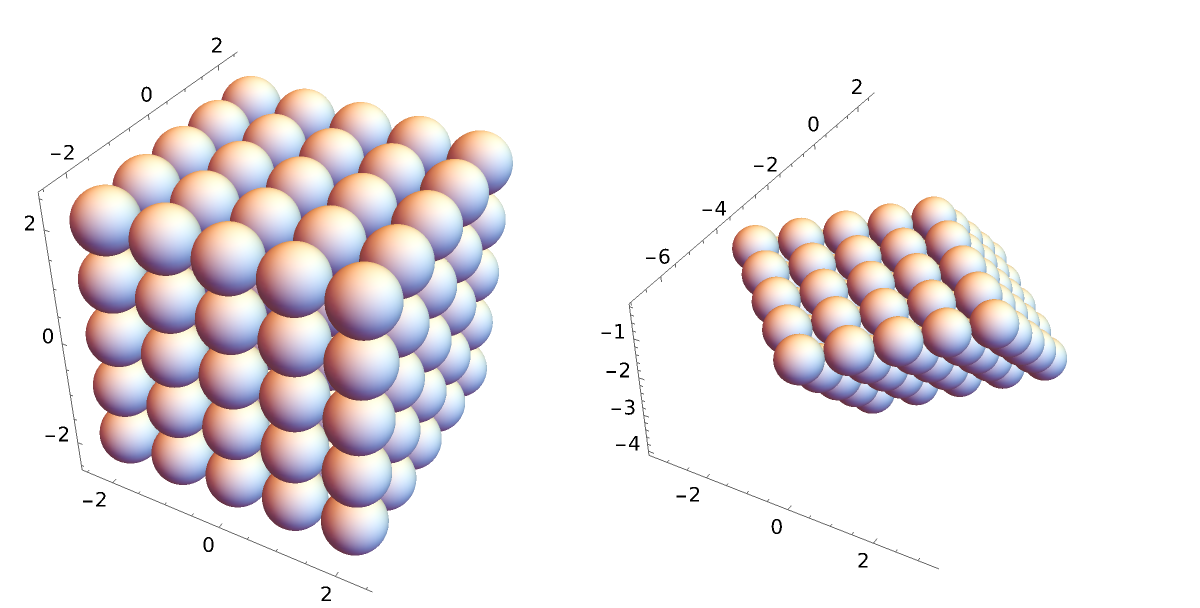Compute and plot the radial distribution functions to compare:

 In:=Out=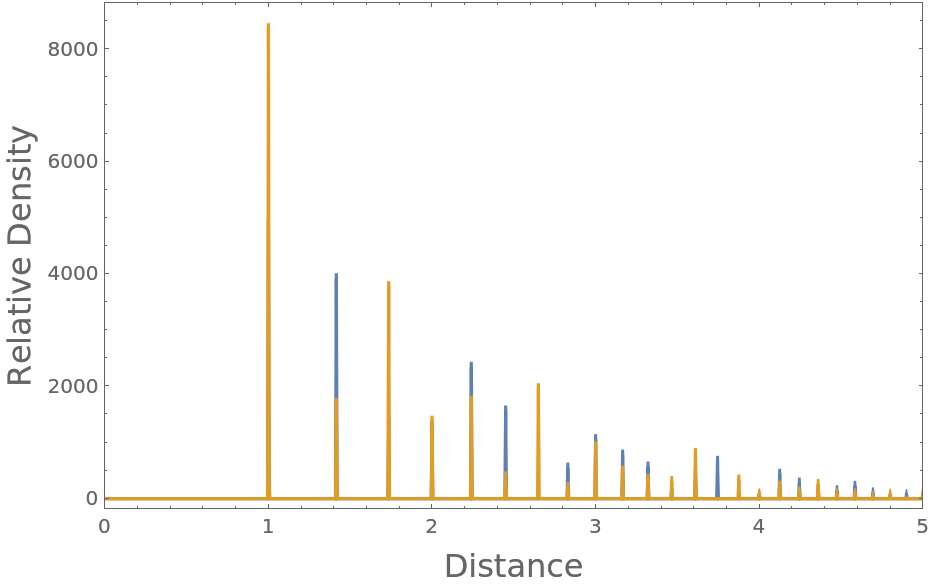Compute the Potential of Mean Force that has given rise to the interactions, in this example showing that the 3D ideal gas has no potential of interaction:

 In:=In:=Out=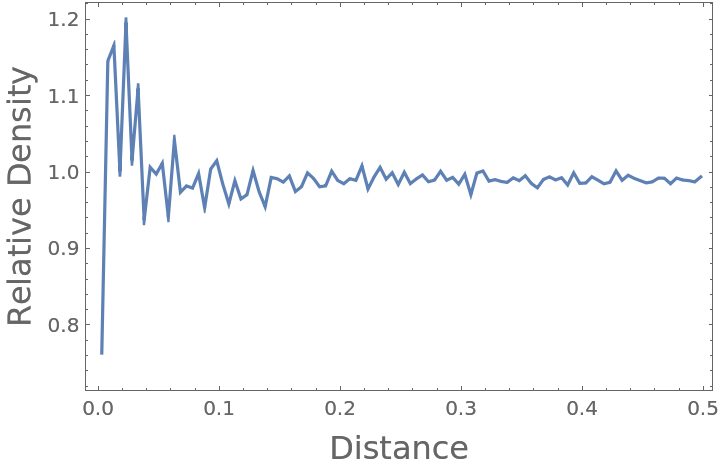Compute the corresponding Potential of Mean Force (in units where kBT=1):

 In:=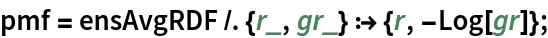In:=Out=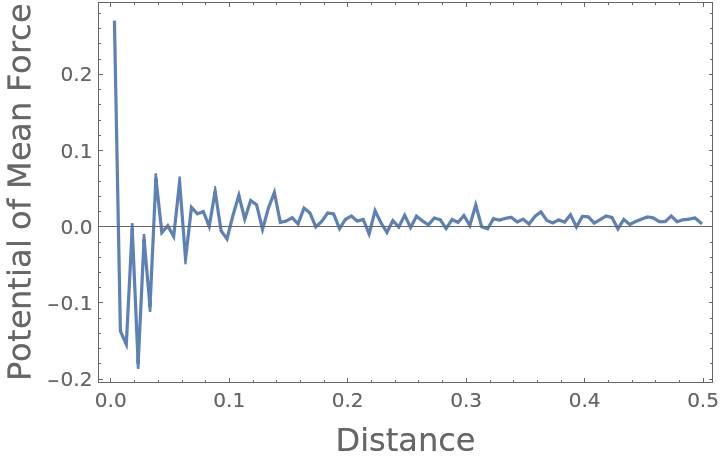It can be seen that despite the noise associated finite data, the Potential of Mean Force is fairly close to zero when averaged over the entire domain:

 In:=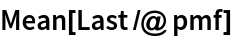Out=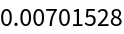## Publisher

Wolfram|Alpha Math Team

## Version History

• 2.0.0 – 23 March 2023
• 1.1.0 – 27 September 2022
• 1.0.0 – 16 September 2020